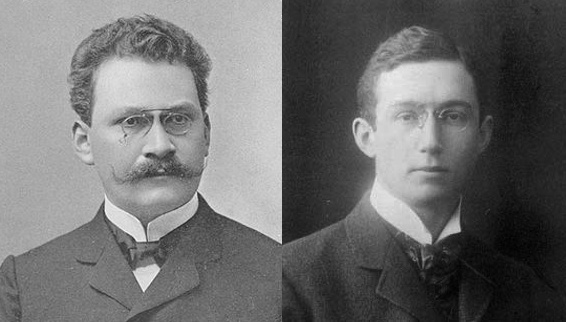### The Yarkovsky Effect

June 12, 2012

The momentum p of a body, as we all learned in our high school physics, is just the product of its mass m and its velocity v,
p = mv
Armed with this classical mechanics result, we are quick to conclude that photons, which carry no mass, should have zero momentum. This is all for the best, since if they had any mass at all, their high speed (the speed of light) would give them a lot of momentum.

Well, classical mechanics is called classical for a reason. When we look at objects outside the limited realm of its postulates, our past wisdom is often not accurate. James Clerk Maxwell's electromagnetic theory shows that electromagnetic radiation, which is the wave complement of particulate photons, will exert a force on objects. Pyotr Lebedev confirmed this experimentally in 1899. Momentum is the integral effect of a force exerted over a time period.An early conception of light having momentum.

A stained glass rendering of the "Fiat Lux" scene of the Bible at the Church of St. Madeleine, Troyes, Aube, France.

(Via Wikimedia Commons))

This radiation pressure, however, is quite feeble. The Sun's radiation will exert a pressure of only 9.2 micro-pascals on a perfectly reflecting mirror on Earth. Feeble though it is, radiation pressure is noticed in the orbits of the GPS satellites, and solar sails have successfully propelled one spacecraft, the Japanese IKAROS spacecraft.

So, what's the momentum of an individual photon? We can calculate it in two different ways,[1-2] one due to Hermann Minkowski, and the other to Max Abraham.

According to Minkowski,
p = h/λ (after de Broglie)
E = hν = hc/λ (after Planck)
λ = hc/E
p = E/c
where λ is the wavelength, h is Planck's Constant, c is the speed of light, and E is energy.

According to Abraham,
E = mc2 (after Einstein)
m = E/c2
p = mv = mc = E/cHermann Minkowski, left, and Max Abraham, right. (Source images. left, right, via Wikimedia Commons.)

Although these calculations give this same result, the calculations presume a vacuum, where the refractive index is one. However, they give different results in a refractive medium, as I reviewed in a previous article (Photon Momentum, March 15, 2007). Even today, a century after these results, physicists are still scratching their heads about this discrepancy.[1-2]

I wrote about negative refractive index materials in a recent article (Mystifying Metamaterials, May 31, 2012). If we use either the Minkowski or Abraham calculation with a negative refractive index, this results in a negative momentum for a photon. A useful result for tractor beams, perhaps?

I mentioned the Yarkovsky effect in a recent article (Asteroid Deflection, April 19, 2012). The Yarkovsky effect is a photon momentum effect with a twist - the photons are emitted by an object, rather than impinging upon it. In this case, a temperature difference of one side of an object versus the other causes an imbalance in its thermal radiation. The differential momentum of radiation emitted from one side of the object versus the other exerts a small force. This anisotropic emission of thermal photons has been proposed as a method of steering asteroids away from a collision with the Earth.[5-6]

The Yarkovsky effect for asteroid deflection isn't just a theory. A 2003 analysis of more than a decade's worth of Arecibo radar observations of the asteroid, 6489 Golevka, allowed a precise calculation of its orbit.[7-8] The asteroid has an irregular shape that leads to Yarkovsky forces (see figure).Radar maps of asteroid 6489 Golevka

(NASA/JPL images, via Wikimedia Commons))

The Yarkovsky force acting on 6489 Golevka is just a quarter newton, but over twelve years of observations, it caused an orbital shift of fifteen kilometers (about ten miles). The authors of the study note that the effect operating on many such asteroids over the course of millions of years would have pushed asteroids in orbits between Mars and Jupiter into near-Earth orbits.[7-8]

As part of a planned asteroid sample return mission by NASA, megalomaniacally named, "Origins, Spectral Interpretation, Resource Identification, Security, Regolith Explorer" (OSIRIS-REx), the orbit of another asteroid, 1999 RQ36, was precisely measured. This measurement is presently the most precise orbit measurement of an asteroid made to date. Radar observations of this asteroid, made at Arecibo in September, 2011, were supplemented with other radar observations in 1999 and 2005 to show that 1999 RQ36 has been influenced by the Yarkovsky effect.

The orbit was measured to an accuracy of 300 meters at a distance of thirty million kilometers (twenty million miles). Asteroid 1999 RQ36 has deviated from its gravity influenced orbit by about 160 kilometers (100 miles) in the last twelve years. The peak Yarkovsky force on the asteroid, when the asteroid was nearest the sun, was only one-eighth of a newton.

### References:

Linked Keywords: Momentum; high school; physics; mass; velocity; classical mechanics; photon; speed of light; axiom; postulate; James Clerk Maxwell; Maxwell's equations; electromagnetic theory; electromagnetic radiation; complementarity; wave complement; particulate; force; Pyotr Lebedev; experiment; integral; time; stained glass; Fiat Lux; Book of Genesis; Bible; Church of St. Madeleine, Troyes, Aube, France; Wikimedia Commons; radiation pressure; Sun; radiation; pressure; pascal; mirror; Earth; GPS satellite; solar sail; Japan; Japanese; IKAROS spacecraft; Hermann Minkowski; Max Abraham; Louis de Broglie; Max Planck; wavelength; Planck's Constant; speed of light; energy; Albert Einstein; vacuum; refractive index; century; physicist; negative index metamaterials; negative refractive index materials; tractor beam; Yarkovsky effect; asteroid deflection; temperature; thermal radiation; anisotropy; anisotropic; asteroid; potentially hazardous object; Earth; Arecibo; 6489 Golevka; orbit; newton; kilometer; mile; near-Earth object; near-Earth orbit; NASA; OSIRIS-REx; 1999 RQ36; radar; meters; Newton's law of universal gravitation.